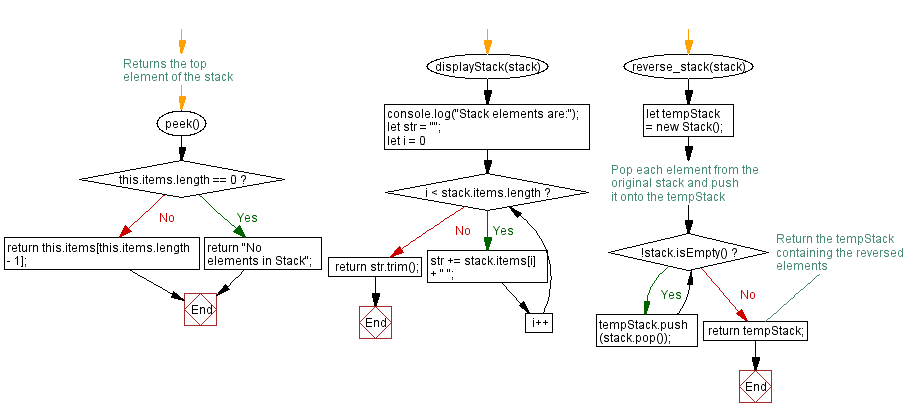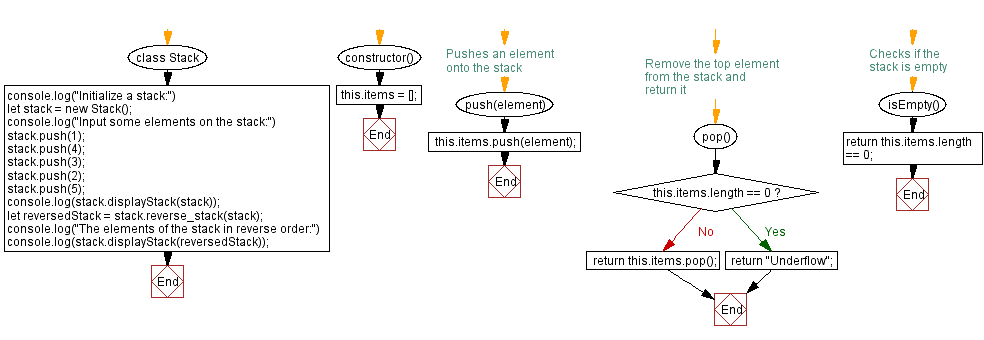# JavaScript Exercises: Reverse the elements of a stack

## JavaScript Stack: Exercise-4 with Solution

Write a JavaScript program to reverse the elements of a given stack.

Sample Solution:

JavaScript Code:

``````class Stack {
constructor() {
this.items = [];
}

// Pushes an element onto the stack
push(element) {
this.items.push(element);
}

// Remove the top element from the stack and return it
pop() {
if (this.items.length == 0)
return "Underflow";
return this.items.pop();
}

// Checks if the stack is empty
isEmpty() {
return this.items.length == 0;
}

// Returns the top element of the stack
peek() {
if (this.items.length == 0)
return "No elements in Stack";
return this.items[this.items.length - 1];
}

displayStack(stack) {
console.log("Stack elements are:");
let str = "";
for (let i = 0; i < stack.items.length; i++)
str += stack.items[i] + " ";
return str.trim();
}

reverse_stack(stack) {
let tempStack = new Stack();

// Pop each element from the original stack and push it onto the tempStack
while (!stack.isEmpty()) {
tempStack.push(stack.pop());
}
// Return the tempStack containing the reversed elements
return tempStack;
}
}
console.log("Initialize a stack:")
let stack = new Stack();
console.log("Input some elements on the stack:")
stack.push(1);
stack.push(4);
stack.push(3);
stack.push(2);
stack.push(5);
console.log(stack.displayStack(stack));
let reversedStack = stack.reverse_stack(stack);
console.log("The elements of the stack in reverse order:")
console.log(stack.displayStack(reversedStack));
```
```

Sample Output:

```Initialize a stack:
Input some elements on the stack:
Stack elements are:
1 4 3 2 5
The elements of the stack in reverse order:
Stack elements are:
5 2 3 4 1
```

Flowchart:Live Demo:

See the Pen javascript-stack-exercise-4 by w3resource (@w3resource) on CodePen.

Improve this sample solution and post your code through Disqus

Stack Previous: Sort the elements of a stack in descending order.
Stack Exercises Next: Find the maximum element in a stack (using an array).

What is the difficulty level of this exercise?

Test your Programming skills with w3resource's quiz.

﻿